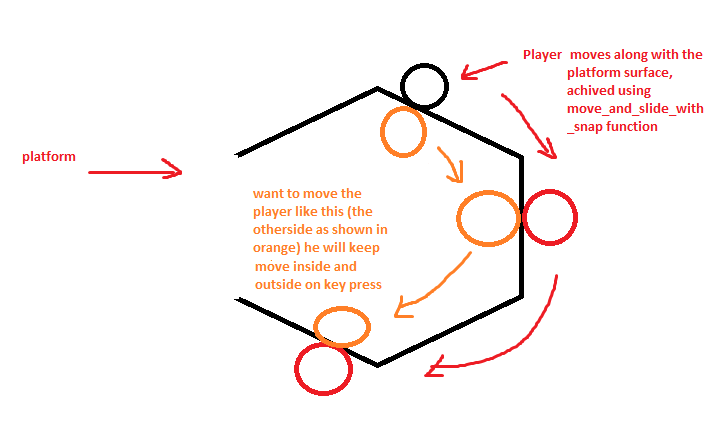Hello guys, I want the player to be moved inside and outside based on keypress. The problem is as the player can be at any angle any time , I'm not able to understand how to move him to some distance inside/outisde.

I have did this

``````snap = transform.y * 128 if !is_jumping else Vector2.ZERO
velocity.y += GRAVITY * delta + force
velocity.x += 1
velocity = velocity.normalized() * SPEED
velocity = move_and_slide_with_snap(velocity.rotated(rotation),snap,-transform.y,true,4,PI/3,false)
velocity = velocity.rotated(-rotation)
``````